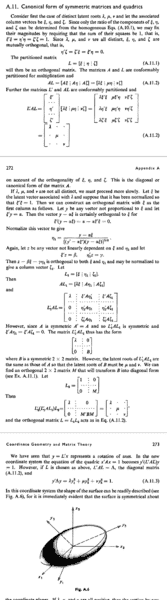# Canonical form derivation of (L1'AL1)

Sanchayan Ghosh
Hello everyone,

I actually had a problem with understanding the part where they have defined L'AL = Λ. There, they have taken
γΛγ1 = Σy2λ = 1. Why have they taken that? Is it arbitary or does it come as a result of a derivation?Thank you

## Answers and Replies

Nguyen Son
sorry, we cannot see the picture

Sanchayan Ghosh
I don't know why it got blurred. Actually I resolved the problem.
y'Δy is nothing but mitiplying the transpose of vector components of y with the symmetric matrix
Δ = L'1AL1
L is the 1x3 matrix of eigen values of a symmetric matrix A.
And then multiplying the result with y vector. This gives the above expression as I had asked.

Thank you.